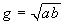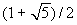www.batmath.it

A Glossary of Mathematical Terms - G

Geometric mean

The geometric mean of two positive numbers a and b is.

Geometric sequence (or progression)

A sequence of numbers where the first one is repeatedly multiplied by a fixed number to obtain the following numbers of the sequence. the fixed number is acalled the common ratio.

Glide reflection

A composite transformation where a figure is reflected and then translated.

Golden rectangle

A rectangle where the ratio of its length to its width is.

See Sphere.

Greatest Common Factor (G.C.F.)

Is the largest common factor betwen two or more numbers, that is the largest numbers that divides all the given numbers.

Grid

A tessellation of congruent squares used in coordinate systems or to measure distances, areas, etc.

first published on september 01 2003 - last updated on september 01 2003